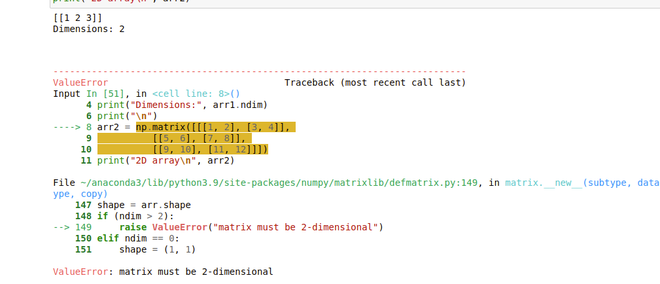Open in App
Not now

# Difference between Numpy array and Numpy matrix

• Difficulty Level : Basic
• Last Updated : 21 Nov, 2022

While working with Python many times we come across the question that what exactly is the difference between a numpy array and numpy matrix, in this article we are going to read about the same.

## What is np.array() in Python

The Numpy array object in Numpy is called ndarray. We can create ndarray using numpy.array() function. It is used to convert a list, tuple, etc. into a Numpy array.

Syntax: numpy.array(object, dtype=None)

Creating a NumPy array using the .array() function.

## Python3

 `# importing numpy module``import` `numpy as np` `# creating numpy array``res ``=` `np.array([``'G'``, ``'F'``, ``'G'``])` `print``(``"Numpy Array in python :"``, res)``print``(``type``(res))`

Output:

```Numpy Array in python : ['G' 'F' 'G']
<class 'numpy.ndarray'>```

## What is numpy.matrix() in Python

A matrix in Numpy returns a matrix from a string of data or array-like object. The matrix obtained is a specialized 2D array.

Syntax: numpy.matrix(object, dtype = None) :

Creating a NumPy matrix using the .matrix() function.

## Python3

 `import` `numpy as np` `mat ``=` `np.matrix([[``1``, ``2``, ``3``],``                 ``[``5``, ``6``, ``7``],``                 ``[``4``, ``6``, ``8``]])` `print``(``"Matrix is : \n"``, mat)``print``(``type``(mat))`

Output:

```Matrix is :
[[1 2 3]
[5 6 7]
[4 6 8]]

<class 'numpy.matrix'>```

## Difference between Numpy array and Numpy Matrix

### Matrix is 2-dimensional while ndarray can be multi-dimensional

Example 1:

Here, we can print all the dimensions of an array in np.array.

## Python3

 `import` `numpy as np` `arr1 ``=` `np.array([``1``, ``2``, ``3``])``print``(``"1D array\n"``, arr1)``print``(``"\n"``)` `arr2 ``=` `np.array([[``1``, ``2``], [``3``, ``4``]])``print``(``"2D array\n"``, arr2)``print``(``"\n"``)` `C ``=` `np.array([[[``1``, ``2``], [``3``, ``4``]],``             ``[[``5``, ``6``], [``7``, ``8``]],``             ``[[``9``, ``10``], [``11``, ``12``]]])``print``(``"3D array\n"``, C)`

Output:

```1D array
[1 2 3]

2D array
[[1 2]
[3 4]]

3D array
[[[ 1  2]
[ 3  4]]

[[ 5  6]
[ 7  8]]

[[ 9 10]
[11 12]]]```

Example 2:

Matrix works normally for a 2D matrix and if a 1D matrix will convert into 2D Matrix, but if we pass a 3D matrix it will through an error.

## Python3

 `import` `numpy as np` `arr1 ``=` `np.matrix([``1``, ``2``, ``3``])``print``(arr1)``print``(``"Dimensions:"``, arr1.ndim)``print``(``"\n"``)` `arr2 ``=` `np.matrix([[[``1``, ``2``], [``3``, ``4``]],``                  ``[[``5``, ``6``], [``7``, ``8``]],``                  ``[[``9``, ``10``], [``11``, ``12``]]])``print``(``"2D array\n"``, arr2)`

Output:### Different functionality of  * operator in ndarray and Matrix

Example 1:

Array * operator does simple multiplication.

## Python3

 `a ``=` `np.array([[``1``, ``2``],``             ``[``3``, ``4``]])``b ``=` `np.array([[``1``, ``2``],``             ``[``3``, ``4``]])` `print``(``"Array multiplication: \n"``, a``*``b)`

Output:

```Array multiplication:
[[ 1  4]
[ 9 16]]```

Example 2:

While it does matrix multiplication.

## Python3

 `a ``=` `np.matrix([[``1``, ``2``],``             ``[``3``, ``4``]])``b ``=` `np.matrix([[``1``, ``2``],``             ``[``3``, ``4``]])` `print``(``"Matrix multiplication: \n"``, a``*``b)`

Output:

```Matrix multiplication:
[[ 7 10]
[15 22]]```

### Matrix has an array.I for inverse, but ndarray has linalg.inv

Example 1:

The inverse can be done with array.I in ndarray.

## Python3

 `arr1 ``=` `np.matrix([[``1``, ``2``],``              ``[``3``, ``4``]])` `print``(``'Inverse \n'``, arr1.I)`

Output:

```Inverse
[[-2.   1. ]
[ 1.5 -0.5]]```

Example 2:

The inverse can be done with np.linalg.inv in matrix.

## Python3

 `b ``=` `np.array([[``1``, ``2``],``             ``[``3``, ``4``]])` `print``(``'Inverse \n'``, np.linalg.inv(b))`

Output:

```Inverse
[[-2.   1. ]
[ 1.5 -0.5]]```

## Table of Differences between the Numpy Array and Numpy Matrix

My Personal Notes arrow_drop_up